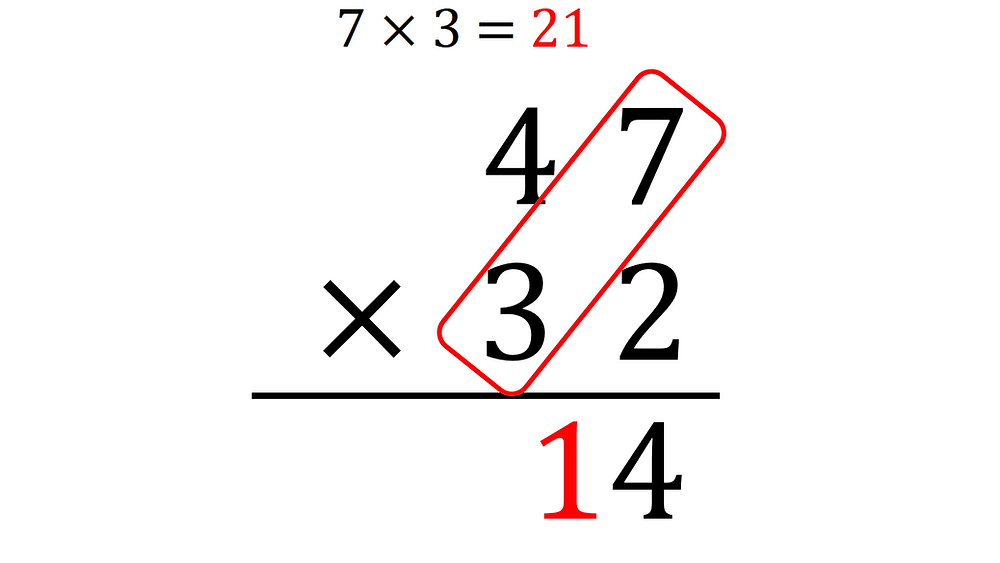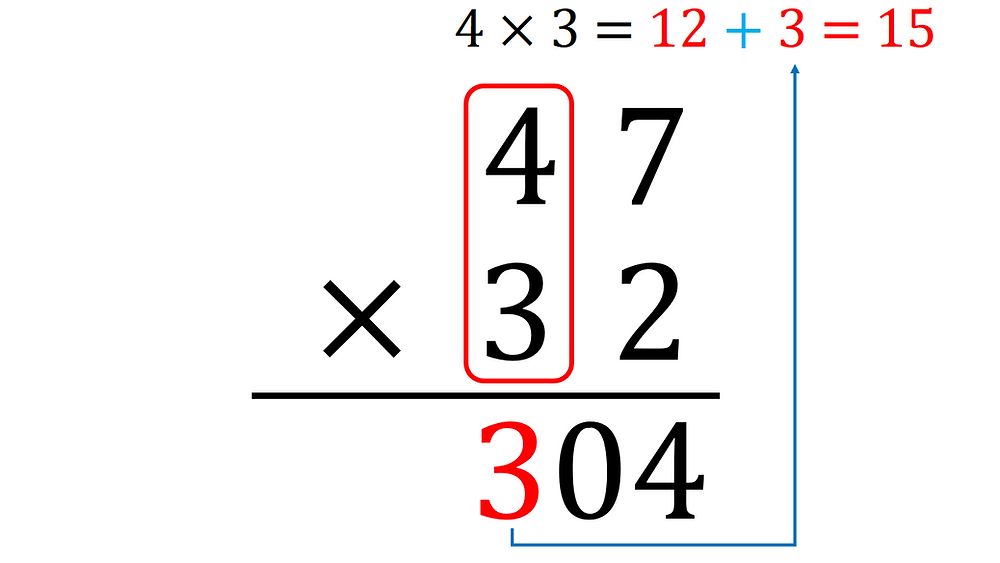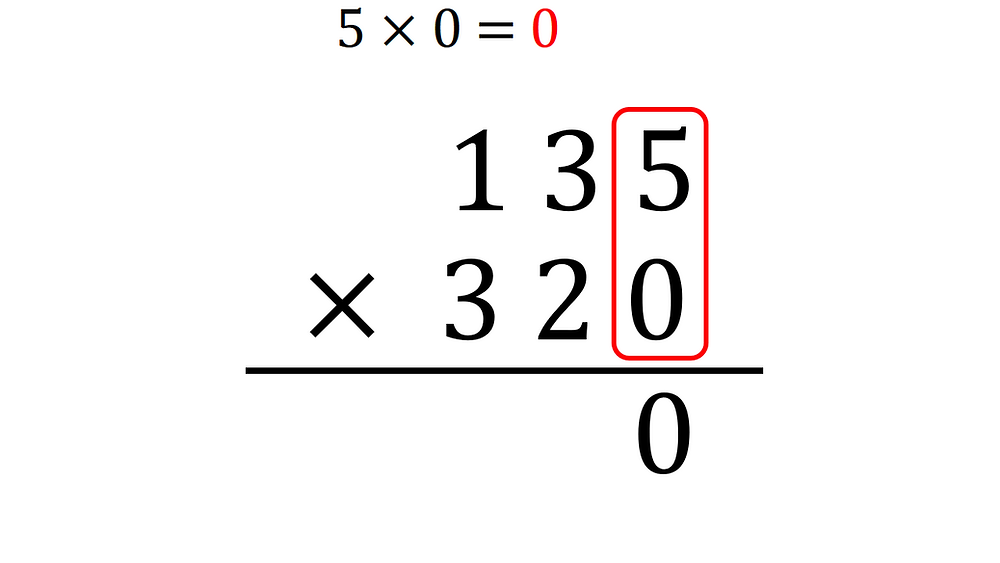Search

# Cross Multiplication Technique: Multiplication Short Trick for Aptitude

### Cross Multiplication method is the easiest and most common method used for multiplication. In Vedic mathematics it is known as ūrdhva tiryagbhyāṃ. It means “Vertically and crosswise.”Let us understand how it works by multiplying 47 X 32:

Step 1: Arrange the number vertically. If the numbers are non-equidistant, then add zeros as prefix before the smaller number to make it equal.Step 2: Multiply the one’s digit together and write the one’s value of the answer below, keeping the ten’s place as carry (I have marked the carry in red).Step 3: Multiply the diagonals and add them together along with the carry value obtained in step 1. Now, write the one’s value of the answer below, keeping the ten’s place as carry (I have marked the carry in red).Step 4: Finally, Multiply the digits in the ten’s digit together and adding the carry from the value obtained in step 2, write the answer to the left of previous value obtained.You may have noticed that the above example was with 2-digit number. Our method only varies slightly if you come across a three-digit number along the way. Here’s an example to demonstrate.

Let us understand how it works by multiplying 135 X 320:

Step 1: Arrange the number vertically. If the numbers are non-equidistant, then add zeros as prefix before the smaller number to make it equal.Step 2: Multiply the one’s digit together and write the one’s value of the answer below, keeping the ten’s place as carry.Step 3: Multiply the diagonals that is formed using the last two digit of each numbers and add them together along with the carry value obtained in step 1. Now, write the one’s value of the answer below, keeping the ten’s place as carry (I have marked the carry in red).Step 4: Multiply the diagonal formed between the one’s place and hundred’s place digit and multiply the ten’s place digit together and then adding them together along with the carry value obtained in step 1. Now, write the one’s value of the answer below, keeping the ten’s place as carry (I have marked the carry in red).Step 5: Multiply the diagonals that is formed using the first two digit of each numbers and add them together along with the carry value obtained in step 1. Now, write the one’s value of the answer below, keeping the ten’s place as carry (I have marked the carry in red).Step 6: Finally, Multiply the digits in the hundred’s digit together and adding the carry from the value obtained in step 2, write the answer to the left of previous value obtained.